# 6 Remarks on Global Solutions

C. W. Misner

#### DOI

10.34663/9783945561294-11

#### Citation

Misner, C. W. (2011). Remarks on Global Solutions. In: The Role of Gravitation in Physics: Report from the 1957 Chapel Hill Conference. Berlin: Max-Planck-Gesellschaft zur Förderung der Wissenschaften.

“Suppose we consider the following set of equations: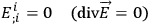6.1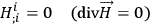6.2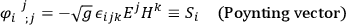6.3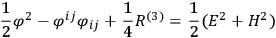6.4

where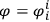and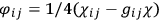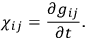We study these equations in order of difficulty. Eq.(6.1) has as its most general solution: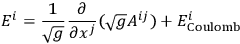6.5

where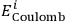has vanishing divergence and also vanishing curl: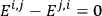6.6

but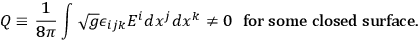6.7

This is only possible if “wormholes” are present. There are as many independent solutions as there are wormholes, and one can specify arbitrarily the charge of each. Eq.(6.3) can now be regarded as an inhomogeneous linear equation. A solution of the inhomogeneous equation exists on a compact manifold if and only if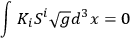6.8

for every Killing vector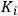(generating a group of motions) satisfying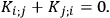6.9

Whether this has any significant applications I do not know. About Eq. (6.4) I can say nothing.

“l conjecture that the homogeneous equation could be solved by the use of potentials, provided we use a rather broad idea of a potential. By this I mean a solution which somehow involves the use of an arbitrary function. To illustrate the idea I give a particular class of solutions to the equation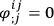, namely: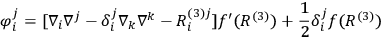6.1

where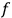is an arbitrary function of the scalar curvature on the surface, and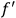is the derivative of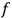.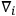is the covariant derivative.

“Besides the problems of topology in the small associated with “flares” and wormholes, or with the symmetric Einstein-Rosen picture, there are the problems of topology in the large. These are familiar problems: Is the universe closed and spherical in a reasonable approximation, or is it open? Other topological problems are the problems of classifying three-dimensional compact manifolds. One can at present write out a list containing all three-dimensional compact manifolds, but one has no way of telling whether two are the same or different by a finite algorithm, since the list may contain redundant cases. Most mathematicians seem to believe that the solution to this problem is not in sight.

“Another point is this: Once you take the course indicated here and decide to make a unified field theory to avoid singularities and you bring in the possibility of non-Euclidean topology, then you would like to know the limitations on the number of topologies that are likely to appear. The problem is not one of finding ingenious enough topologies to do what you want them to do, but rather to find a reason why a host of topologies will never appear. One must examine the 4-dimensional manifold. Professor Lichnerowicz has mentioned that for most manifolds which are not of the type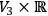there will not exist reasonable space-like surfaces. However, the limitation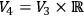might be too restrictive. In other words: Can the number of particles in a universe with wormholes change? For instance, one might ask if a wormhole can disappear in time. Can it happen that starting from a wormhole topology at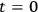you arrive at a Euclidean space at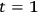?

Fig. 6.1

From the work of Thom1 one can show that there are four-dimensional manifolds which have these two surfaces as boundaries. The question whether a continuous metric of Lorentz signature can be put on such manifolds can, I believe, be answered. In such a metric can you find solutions to any hyperbolic equations, or better, Maxwell’s equations? Anyone trying to study this would be much aided by certain qualitative classifications of metrics. I conjecture that if you divide the metrics which it is possible to put on a given manifold whose two boundaries are space-like, into homotopy equivalence classes, then ordinarily there will not be more than one of these classes which allows any solutions to a hyperbolic equation; i.e., most homotopy classes are inconsistent with hyperbolic differential equations.”

MISNER concluded with a few remarks about local invariants, such as those given by Géhéniau2 and others: “Instead of the string of components of the Ricci tensor, one looks at all the invariants which can be constructed from these. One possible use of these might be as variables in discussing the quantization of general relativity, since quantization seems to handle invariant quantities better than tensor components. Also it would be interesting to see Gedankenexperiment to measure each of these curvature invariants. This would be a beginning for making simple quantum mechanical arguments about measurability in general relativity. Perhaps Pirani could give a characterization of pure gravitational radiation in terms of these invariants?”

BERGMANN pointed out that in the study of the equations of general relativity today one seems to have a choice of obtaining either general local properties or special global theorems.

MME. FOURES then gave her solution of the problem of the initial values using Cartan’s exterior differential calculus:

## Footnotes

Comment Math. Helv. 28, 17-86 (1954), Theorem IV, 13. Also see B. A. Rokhlin, Doklady Akad. Nauk. SSSR 81, 355 (1951).

J. Géhéniau and R. Debever, Bull. Cl. des Sci., Acad. roy. Belg.,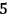Sér., 42, (1956).